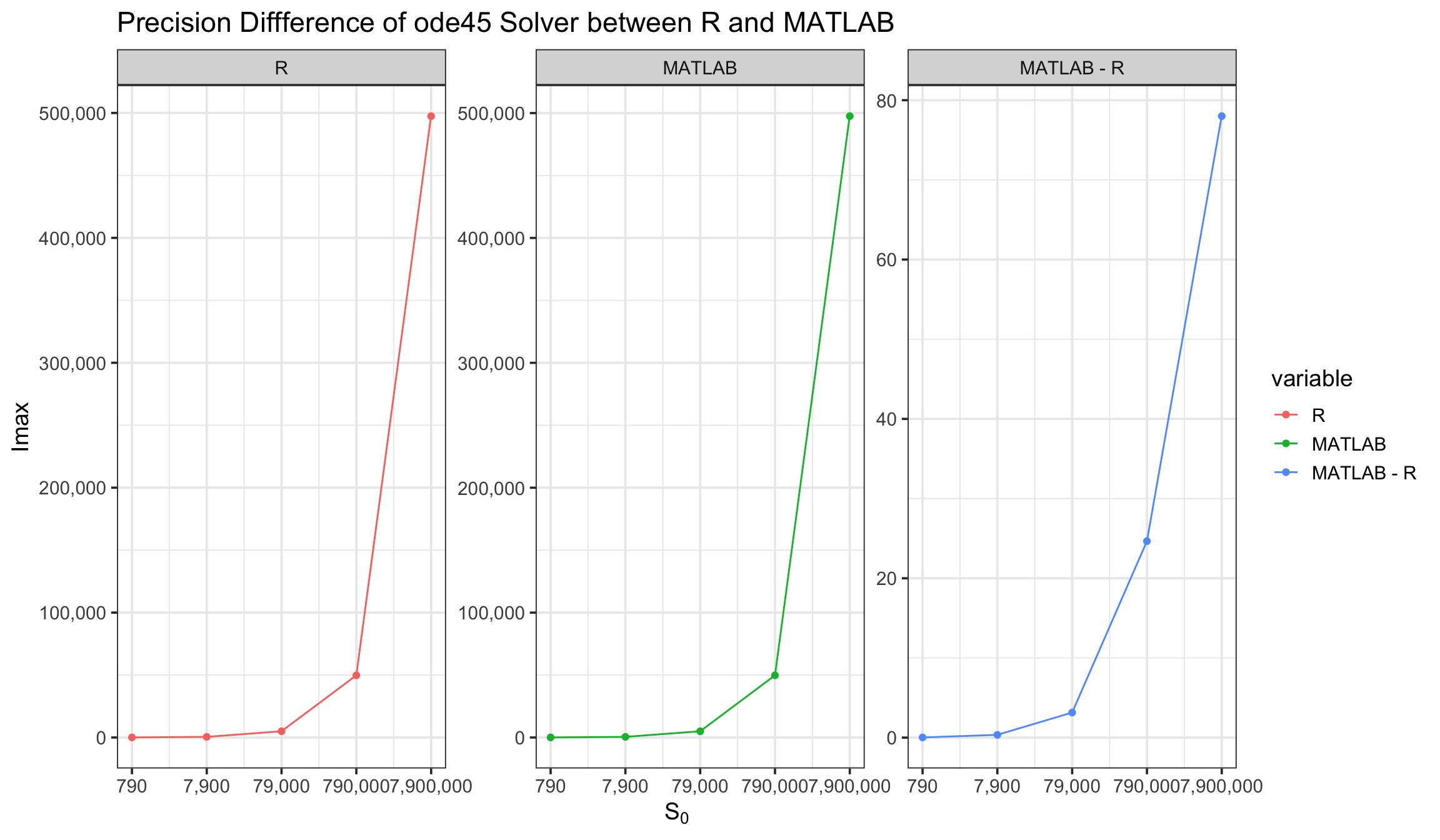# Tony Tsai

## May the force of science be with you

Nov 24, 2014 - 1 minute read - Comments - Numerical Computation

# Precision Difference of ode45 between R and MATLAB

ode45 solvers from both R and MATLAB are used to run simulations of the spread of Hong Kong flu in New York city with different initial susceptible individuals $S\_0 =$ 790, 7900, 79000, 790000, and 7900000. The maximum infected people $I_{max}$ under each condition is recorded for comparison. The following codes draw a figure showing the precision difference of ode45 solvers between R and MATLAB.

Imax <- data.frame(S0 = 79 * 10 ^ (1:5),
R = c(57.12598, 504.9923, 4984.303, 49749.60, 497474.2),
MATLAB = c(57.14987, 505.3385, 4987.447, 49774.26, 497552.2))
Imax$MATLAB - R <- Imax$MATLAB - Imax\$R
# plot delta Imax VS. S0
library(reshape2)
Imax.plot <- melt(Imax, id = "S0", measure = c("R", "MATLAB", "MATLAB - R"))
library(ggplot2)
library(scales)
p <- ggplot(Imax.plot, aes(x = S0, y = value, group = variable, color = variable))
p + geom_line() +
geom_point() +
scale_x_log10(name = expression(S), breaks = 79 * 10 ^ (1:5), labels = comma) +
scale_y_continuous(name = "Imax", labels = comma) +
facet_wrap(~ variable, scales = "free") +
ggtitle("Precision Diffference of ode45 Solver between R and MATLAB") +
theme_bw(base_size = 14)Tags: ode45 R MATLAB